Deutsche VersionCalculation: Amplification (gain) and damping (loss)
as
factor ( ratio) to the level in decibels (dB)

 Gain is the ratio between the magnitude of output and input signals. Gain controls on an amplifier are basically just small potentiometers (variable resistors) or volume controls, that allow you to adjust the incoming signal to the amplifier.

The amplification factor, also called gain, is the extent to which a device boosts the strength of a signal.
The damping factor, also called loss, is the extent to which a device reduces the strength of a signal.

Enter two values and press the right calculate bar in the line of the missing answer

 Input: voltage power Input value 1 Output value 2 Level change dB
In analog audio engineering we deal only with 'voltage' amplification (gain) and damping (loss).V1 = Vin and V2 = Vout V2 > V1 or Vout > Vin means amplification. The dB value is positive (+). V2 < V1 or Vout < Vin means damping. The dB value is negative (−). V2 / V1 or Vout / Vin means the ratio. The amplification or the damping in dB is: L = 20 × log (voltage ratio V2 / V1) in dB.        V1 = Vin is the reference.

In physics, attenuation is regarded as a positive value.
This naturally leads to sign errors when entering numbers.

 3 dB ≡ 1.414 times the voltage (−)3 dB ≡ damping to the value 0.707 6 dB ≡ 2 times the voltage (−)6 dB ≡ damping to the value 0.5 10 dB ≡ 3.162 times the voltage (−)10 dB ≡ damping to the value 0.316 12 dB ≡ 4 times the voltage (−)12 dB ≡ damping to the value 0.25 20 dB ≡ 10 times the voltage (−)20 dB ≡ damping to the value 0.1

 Using voltage we get: Level in dB: L = 20 × log (voltage ratio)

 6 dB = twice the voltage 12 dB = four times the voltage 20 dB = ten times the voltage 40 dB = hundred times the voltage

If we consider audio engineering, we are usually not interested in power.
Do not ask what power amplification means.
Leave that to the telephone companies or the transmitting aerials (antennas).
Power gain is really not used in audio engineering.
Do we really need power (energy) amplification?
Read the text at the bottom.

 3 dB ≡ 2 times the power (−3) dB ≡ damping to the value 0.5 6 dB ≡ 4 times the power (−6) dB ≡ damping to the value 0.25 10 dB ≡ 10 times the power (−10) dB ≡ damping to the value 0.1 12 dB ≡ 16 times the power (−12) dB ≡ damping to the value 0.0625 20 dB ≡ 100 times the power (−20) dB ≡ damping to the value 0.01

Using power we get: Level in dB: L = 10 × log (power ratio)

 3 dB = twice the power    6 dB = four times the power  10 dB = ten times the power  20 dB = hundred times the power

If you search for the amplification ratio, given the dB value,
then go to the program dB calculation

Amplification (Gain) and Damping (Loss)

 To use the calculator, simply enter a value. The calculator works in both directions of the ↔ sign.

 Gain ratio v = V2/V1 for field quantities, e.g. voltage ↔ Amplification level L (gain)   voltage level dB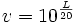Voltage gain v = 1 ≡ 0 dB

In audio technique the following "power or energy amplification " is rather unusual.

 Gain ratio v = P2/P1  for energy quantities, e.g. power ↔ Amplification level L (gain)   power level dB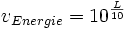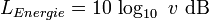Power gain v = 1 ≡ 0 dB
 Voltage/Pressure    amplification ratio 1 1.414  = √2 2 3.16 = √10 4 10 20 40 100 1000 Increasing of x dB 0 3 6 10 12 20 26 32 40 60

 Power/Intensity     amplification ratio 1 1.414  = √2 2 3.16 = √10 4 10 20 40 100 1000 Increasing of y dB 0 1.5 3 5 6 10 13 16 20 30At the cut-off frequency fc the dropped voltage is always fallen to the value of 1/√2 = 0.7071 (70.7%) and the voltage level L is damped to 20 × log10 (1/√2) = (−)3.0103 dB.    At the cut-off frequency fc the dropped power is always fallen to the value of 1/2 = 0.5 (50%) and the power level L is damped to 10 × log10 (½) = (−)3.0103 dB.The expressed voltage gain in dB (voltage amplification) at the cutoff frequency fc is 20 × log10 (1/√2) = (−)3.0103 dB less than the maximum voltage gain.   The expressed power gain in dB (power amplification) at the cutoff frequency fc is 10 × log10 (½) = (−)3.0103 dB less than the maximum power gain.

 To use the calculator, simply enter a value. The calculator works in both directions of the ↔ sign.

 Voltage V   volts ↔ Voltage level LU  dBV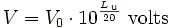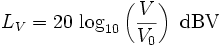Reference voltage V0 = 1 Volt ≡ 0 dBV
 Voltage V (Audio)  volts ↔ Voltage level LU (Audio) dBu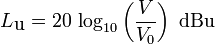Reference voltage V0 = 0.7746 Volt ≡ 0 dBu

The voltage is always given as RMS value - but that is not valid for electric power.

 Electric power P   watts ↔ Electric power level LP  dBReference electric power P0 = 1 W ≡ 0 dB

There is also the reference power P0 = 1 milliwatt or 0.001 watt ≡ 0 dBm

 Electric power P (Telephone)   watts ↔ Electric power level LP dBmReference electric power P0 = 1 milliwatt = 0.001 watt ≡ 0 dBm

Level in psycho acoustics as subjectivly perceived loudness (volume)
The vague human feeling of the double loudness (volume) is specified
with about 6 to 10 dB. This personal feeling is not an exactly measurable value.

Conversion: Gain G, Voltage ratio AV, and Power ratio AP
 Gain G dB | Voltage ratio (factor) AV | Power ratio (factor) AP | | ↓ | ↓ | ↓ | | Voltage ratio (factor) AV | Voltage gain GV dB | Power gain GP dB Power ratio (factor) AP | |
 Voltage gain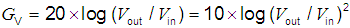in dB Power gain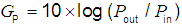in dB Voltage ratio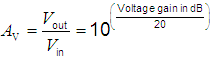= amplification factor (voltage) Power ratio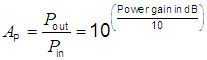= amplification factor (power)V1 = Vin and V2 = Vout. V2 > V1 or Vout > Vin means amplification. The dB value is positive. (+) V2 < V1 or Vout < Vin means damping. The dB value is negative. (−) V2 / V1 or Vout / Vin means the ratio. The amplification or the damping in dB is: L = 20 × log (voltage ratio V2 / V1) in dB.        V1 = Vin is the reference.The word "power amplifier" is a misnomer - especially in audio engineering. Voltage and current can be amplified. The strange term "power amplifier" has become understood to mean an amplifier that is intended to drive a load such as a loudspeaker. We call the product of current gain and voltage gain "power amplification".